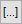Multiple Attributes and Objective Functions

#Multiple Attributes and Objective Functions

A simple DPL model uses only one attribute to compare decision alternatives to determine which is optimal. This is fine if you are concerned with only one measure, say Cost, to determine the optimal policy. But what if you want to find the best policy considering both Cost and Schedule?

DPL handles such multi-attribute problems easily. You have two options: you can combine the attributes in the value model, or you can track each attribute separately and combine them using an objective function.

For information on how to specify attributes and objective functions, see Objective & Utility dialog

Using Multiple Attributes in Get/Pay expressions

Once you have created multiple attributes, every get/pay expression in the tree must include as many values as there are attributes. The values are separated by commas. For example, a get/pay expression on a branch in the tree of a two-attribute model might be

`Repair_Cost, Days_Of_Delay`

If only one of the attributes is affected when a get/pay expression appears, use a 0 as a placeholder for the others. This might occur, for example, if Cost and Schedule depend on different events.

You can enter expressions for each attribute in the Get/Pay tab of the Branch Definition dialog. There are a number of ways to do this. If you have separate value nodes or other distinct expressions for each attribute, simply enter each expression in the appropriate row on the Get/Pay tab.

If you have multidimensional value nodes (arrays or series), you can define the get/pay expressions so that they are elements of a particular value node. For example, you might have a 1 by 5 import value node called Profits, which you want to Get at the end of the tree. In this situation, you would select the Profits variable from the list for the second attribute (after the objective function) and then click the Fill Down button. DPL will fill in the elements of this array to define the get/pay expressions.

The procedure for using the Fill Down button is similar for other types of series or arrays. For example:

A) Suppose you have defined a 5x1 column vector (array) called Profits_5x1. Just as with the row vector, you would select Profits_5x1 from the variables list and enter it for Attribute2. The Fill Down button will then fill in the other four attributes with elements of the column vector, which are denoted Profits_5x1, Profits_5x1, etc.

B) Suppose you have defined a 2x5 (two-dimensional) array called Profits_2x5. As with the one-dimensional arrays, you would select Profits_2x5 for Attribute2, and use the Fill Down button. In this case DPL will ask you whether to fill in with the first row or the first column of the array.

C) You can also start filling in from an element of an array other than the first element. To do this, enter, for example, Profits_1x10 for the first attribute you wish to fill in, and again use the Fill Down button to fill in the subsequent array elements. In this example, DPL would fill in array elements 6 through 10 for the five attributes.

The Objective Function

In the objective function, you refer to the attributes by using the attribute name. In the first example below, you are telling DPL to multiply the second attribute by 100,000 before adding it to the first attribute. This could reflect an estimate that every unit of time costs you 100,000 in addition to the actual cost of the repairs.

The following are examples of objective functions:

• `Attribute1 + Attribute2 * 10000`
• `Attribute1 * Cost1 + Attribute2 * Cost2`
• `Attribute1 / Attribute2`

The second objective function listed above is Attribute1 times Cost1 plus the Attribute2 times Cost2. Cost1 and Cost2 can be values or named constants. If they are values, they cannot depend on the states of any events.

The third objective function divides the Attribute1 by Attribute2. This might be appropriate in a decision problem in which the first attribute measures benefit and the second attribute measures cost. The objective function produces a cost-benefit ratio.

You do not have to include all attributes in the objective function. When there is only one attribute, DPL's default objective function is equal to that attribute. (When there is more than one attribute, the default objective function is the sum of the attributes) However, you can specify an expression for the objective function even where there is only one attribute. This allows you to multiply all the gains and losses by a scale factor before DPL uses them in an analysis. It also lets you use any expression to transform the sum of the gains and losses before applying a utility function (if any) and determining the optimal policy.

In some cases, you might want to break a single composite attribute into several individual attributes to get some extra information about the way your model is working. For example, if your output model calculates costs that include contributions from various sources (for example, costs for marketing and costs for distribution), you can collect them as separate attributes and then combine them to form total costs in your objective function. You could then get distributions on each cost and compare them.

Keeping track of multiple attributes can increase the execution time required for your analysis. If you are only interested in the optimal Policy Tree, and not the probability distribution for each attribute, you can specify any decision problem with a single attribute. You can do so by using the expressions that represent gains or losses for each event state to combine any attributes into a single value. However, this approach has the disadvantage that it is easier to modify an objective function if it appears only once in a program rather than in expressions defining gains and losses throughout a decision tree.

Versions: DPL Professional, DPL Enterprise, DPL Portfolio